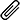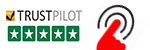High Quality, Fast Delivery, Plagiarism Free - Just in 3 Steps

## Upload Questions Details and Instructions:Select Assignment Files

## Get Assignment Help by Experts

### Plagiarism-Free, Every Time

All of our assignment solution delivers with a dedicated plagiarism report

### Excellent Subject Matter Expert

Get Supreme Assignment Help by highly Skilled Writers

### 100% Satisfaction Guaranteed

Score Higher or Your Money Back# MATLAB Programming Assignment on Engineering Simulations and Computations 1,2

## Q 1    (worth 115 marks)

1.1    Introduction

Further analysis of the viaduct from Assignment 3 Question 3 is to be performed. An important reason for the construction of the Range Crossing is to reduce the gradient of the road for trucks. You will analyse the data in ass4q1in.csv to calculate gradients of the road with and without the viaduct. For this question, we are assuming that the viaduct follows a straight line between its end-points and the x-coordinate has been reoriented to act along the length of the bridge.

1.2    Requirements

For this assessment item, you must perform hand calculations:

1. Assuming that there is no viaduct, estimate the value of the gradient ∂z/∂x at the second measurement point using backward, forward and central differences.

2. Discuss which is the best result out of the backward, forward and central differences and why.

3. Estimate the overall gradient of the viaduct. You must also produce MATLAB code which:

4. Calculates backward, forward and central differences for ∂z/∂x for the length of the road, assuming there is no viaduct. The calculations should be for each possible section (you will not calculate differences for every value of x).

5. Verifies the results from Requirement 4 using the results of Requirement 1.

6. **Estimates the numerical error in the calculations of Requirement 4. Perform this using numerical derivatives for as many data points as possible; at the ends of the data, use the value closest to the end (e.g. for the 1st datum, use the second derivative calculated at the 2nd datum, and the third derivative calculated at the 3rd datum).  For data spaced evenly in x, the necessary formulae are [the methods are O(∆x2) accurate]:

For data unevenly  spaced in x, the necessary formulae are [the methods are O(∆x) accu- rate]:

7. Plots the results from Requirements 4 and 6. Also plots ∂z/∂x (correct Requirement 4 us- ing Requirement 6) together with the central difference from Requirement 4. On the plots of the gradient, also show the viaduct average gradient. Discusses the results, including which is the best method.

1.3    Assessment Criteria

Your code will be assessed using  the following scheme. Note that you are marked based on how well you perform for each category,  so the correct answer determined in a basic way will receive half marks and the correct answer determined using an excellent method/code will receive full marks.

 Quality of hand calculations 30 marks Quality of difference calculations 30 marks Quality of verification 5 marks Quality of error calculations 30 marks Quality of the plots 10 marks Quality of the discussion 5 marks Quality of header(s) and comments 5 marks

We're here to instantly help! Get best assignment questions and answers help 24/7 and Earn better grades with homework